Tailoring univariate probability distributions

By | June 26, 2014

This post shows how to build a custom univariate distribution in R from scratch, so that you end up with the essential functions: a probability density function, cumulative distribution function, quantile function and random number generator.

In the beginning all you need is an equation of the probability density function, from which everyting else can be derived sometimes analytically, and always numerically. The analytical solutions may require some math skills, or may be impossible to find, but the numerical solutions are always feasible, require no math literacy, and coding them in R is easy with the uniroot() and integrate() functions.

Throughout the post I will use a simple exponential distribution as an example.

Probability density function (PDF)

You will need to know what probability density (or mass) function is, and what is the difference between probability density and probability. I found the best intro to be this Khan's video (10 min). Here is a couple of additional points:

• Probability density is relative probability.
• Probability density function evelauated for a given data point is the point's likelihood.
• Probability density can be greater than one.

Example: Exponential PDF

The formula for the exponential probability density function (PDF) is:

In literature, small $p$ usually denotes probability density, while capital $P$ is used for probability.

We can code it in R:

  my.dexp <- function(x, lambda) lambda*exp(-lambda*x)
my.dexp(x=0:5, lambda=2)

##  2.0000000 0.2706706 0.0366313 0.0049575 0.0006709 0.0000908


And compare it with R's native PDF (it uses rate instead of $\lambda$):

  dexp(x=0:5, rate=2)

##  2.0000000 0.2706706 0.0366313 0.0049575 0.0006709 0.0000908


Here is a plot of my PDF using the R's built-in function curve():

  curve(my.dexp(x, lambda=2), from=0, to=2, main="Exponential PDF")Cumulative distribution function (CDF) - analytical solution

This function gives, for a given point $x$, the area under the PDF curve all the way down to the left of the point $x$. This area is the probability that an outcome $X$ will have value lower or equal to $x$:

I liked this stepbil's video (9 min) that shows how to flip between PDF and CDF, and why do we need the $\pi$ in the integral. I am really lame with calculus and what really works for me is Sage – it is free, it finds analytical solutions of integrals, and it may be worth a shot before diving into numerical integration.

For the exponential distribution the CDF is:

In R it is:

  my.pexp.anal <- function(x, lambda) 1- exp(-lambda*x)
my.pexp.anal(x=0:5, lambda=2)

##  0.0000 0.8647 0.9817 0.9975 0.9997 1.0000


Cumulative distribution function (CDF) - numerical solution

For most practical purposes, numerical solutions of one- or two- dimensional problems seem to be as good as the analytical solutions, the only disadvantage is the computational demands. Here I used the R's native function integrate() to calculate the exponential CDF numerically:

  my.pexp.numerical <- function(x, lambda)
{
# this my.int function allows my CDF to run over vectors
my.int <- function(x, lambda) integrate(my.dexp, lambda=lambda, lower=0, upper=x)$value # apply the my.int to each element of x sapply(x, FUN=my.int, lambda) } my.pexp.numerical(x=0:5, lambda=2)  ##  0.0000 0.8647 0.9817 0.9975 0.9997 1.0000  And let's plot the numerical solution over the analytical one:  curve(my.pexp.anal(x, lambda=2), from=0, to=2, col="green", main="exponential CDF") curve(my.pexp.numerical(x, lambda=2), from=0, to=2, lty=2, col="red", add=TRUE) legend("bottomright", lty=c(1,2), legend=c("Analytical", "Numerical"), col=c("green", "red"), lwd=2)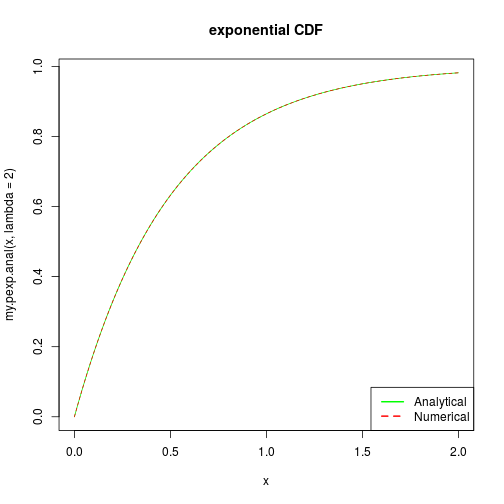Practically identical. Quantile function (QF) - analytical solution Quantile functions are useful for two things: (1) assessing statistical significance, and (2) generating random numbers (see below). Quantile function ($P^-$) is the inverse of the cumulative distribution function. It means that you have to find a value of $x$ for a given $P(x)$, which is an inverse action to what we usually do with functions. Finding an analytical solution may be quite tricky, and again, if you don't have a mathematician at your service, Sage can help. In case of our exponential distribution, the quantile function is: In R:  my.qexp.anal <- function(q, lambda) (-log(1-q))/lambda my.qexp.anal(0.9, 2)  ##  1.151  Quantile function (QF) - numerical solution The inverse may be hard to get for many CDFs, and hence one may need to go for a numerical solution. I found that the easiest way to solve for inverse of univariate CDFs in R is the uniroot() function. Alternatives are optimize() or optim(), but they can be unstable. In contrast, uniroot() is simple, quick, and stable. Here is my numerical solution for the expoentnial QF:  my.qexp.numerical <- function(q, lambda) { # function to be solved for 0 f <- function(P, fixed) { lambda <- fixed$lambda
q <- fixed$q # this is the criterion to be minimized by uniroot(): criterion <- q - my.pexp.numerical(P, lambda) return(criterion) } # for each element of vector P (the quantiles) P <- numeric(length(q)) for(i in 1:length(q)) { # parameters that stay fixed fixed <- list(lambda=lambda, q=q[i]) # solving the f for 0 numerically by uniroot(): root.p <- uniroot(f, lower=0, upper=100, # may require adjustment fixed=fixed) P[i] <-root.p$root
}
return(P)
}

my.qexp.numerical(0.9, 2)

##  1.151


Let's plot the numerical solution over the analytical one:

  q <- seq(0.01, 0.9, by=0.01)
plot(q, my.qexp.anal(q, lambda=2), type="l", col="green", lwd=2)
lines(q, my.qexp.numerical(q, lambda=2), col="red", lty=2)
legend("bottomright", lty=c(1,2),
legend=c("Analytical", "Numerical"),
col=c("green", "red"), lwd=2)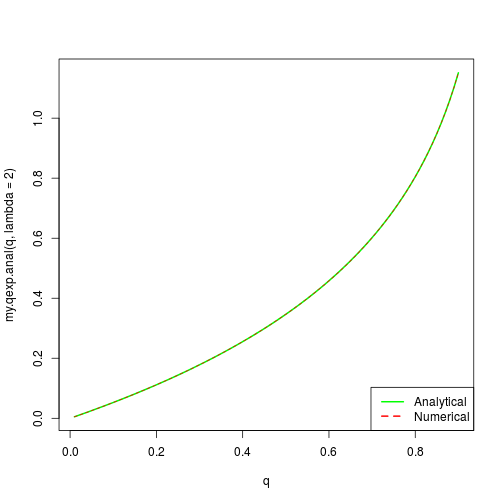Random number generator - the inverse transform sampling

Have you ever wondered how R generates random draws from its distributions? How does it convert uniformly distributed pseudo-random numbers to, e.g., normally distributed numbers? For some time I naively thought that there are some giant built-in tables that R uses. Then I found that it's really simple.

The easiest way is the inverse transform sampling. It works like this: you generate a random number from $Uniform(0,1)$ and plug it into your quantile function (see above). That's it, and that's also why quantile functions can be really useful. Here is how you do it in R with the exponential distribution:

  my.rexp.inverse <- function(N, lambda)
{
U <- runif(N, min=0, max=1)
rnd.draws <- my.qexp.anal(U, lambda)
return(rnd.draws)
}
my.rexp.inverse(10, 2)

##   0.10087 0.56874 0.79258 0.01962 1.28166 0.39451 2.17646 0.48650
##   0.97453 0.33054


Here is a histogram of 10,000 random numbers generated by the inverse transform sampling. The solid smooth line is the exponential PDF from which the random numbers were drawn:

  hist(my.rexp.inverse(10000,2), freq=FALSE, breaks=20,
xlab="x", main=NULL, col="grey")
#hist(rexp(10000, rate=2), freq=FALSE, breaks=20)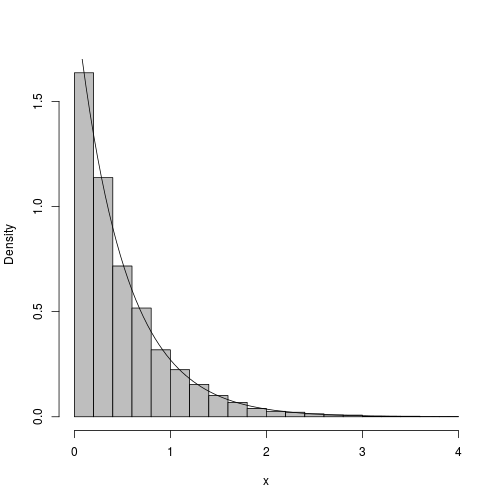Random number generator - rejection sampling

The advantage of rejection sampling is that you don't need the quantile function.
Notable texts on rejection sampling are provided by Jay Emerson, on Quantitations blog and on tsperry's blog. I will try to provide my own description and notation, which will hopefully complement the other posts.

In short, wou will need: (1) the so-called proposal probability densidy function which I will call $f(x)$, (2) the PDF from which you want to sample, here called $p(x)$, and (3) a constant $m$ which will satisfy $f(x) \times m > p(x)$. In other words, the curve $f(x) \times m$ must lay entirely above the $p(x)$ curve, and ideally as close to $p(x)$ as possible (you will waste less samples). The $f(x)$ can be any PDF from which you are able to sample.

For my exponential example I will use simple uniform proposal density $f(x)$. The following figure illustrates my exponential $p(x)$, my proposal $f(x)$, and $f(x) \times m$:

  x <- seq(0, 5, by=0.01)
# my exponential PDF:
p <- dexp(x, rate=2)
# my PROPOSAL distribution:
f <- dunif(x, min=0, max=5) # my proposal is uniform and hence
# I need to choose an arbitrary upper bound 5
# the CONSTANT m
m <- 11
fm <- f*m

plot(c(0,5), c(0,2.5), type="n", xlab="x", ylab="density")
lines(x, fm, type="l", col="red")
lines(x, f, col="red", lty=2)
lines(x, p)

legend("right", lty=c(1,2,1),
legend=c("f(x)*m", "f(x)", "p(x)"),
col=c("red", "red", "black"), lwd=2)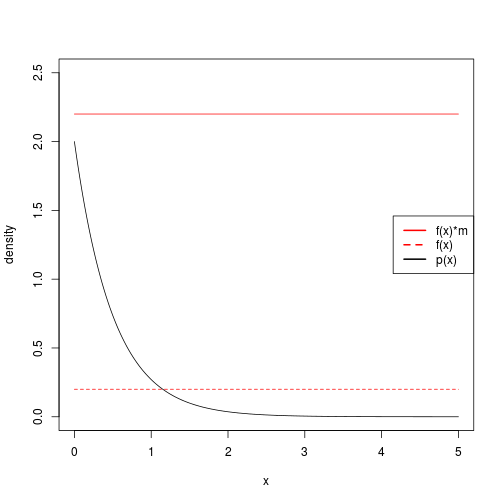My uniform propsal distribution is not optimal – it will lead to high rejection rate and will require a lot of unnecessary computations. The uniform proposal will also truncate my samples at the max value of the uniform distribution. However, the uniform will still be ok for the illustration purposes here.

In rejection sampling the algorithm of one sampling step is:

• 1. Sample a point $r_x$ from $f(x)$.
• 2. Draw a vertical line from $r_x$ all the way up to $m \times f(x)$.
• 3. Sample a point $r_v$ from a uniform density along the vertical line.
• 4. If $r_v \leq p(x)$ accept the sample, otherwise go to 1.

This step is repeated until the desired number of accepted samples is reached.

Here is my rejection sampler for the exponential PDF:

  my.rexp.reject <- function(N, lambda, max)
{
samples <- numeric(N)
m <- 12
fxm <- dunif(0, 0, max)*m
for (i in 1:N)
{
repeat
{
# 1. sample from the propsal distribution:
rx <- runif(1, 0, max)
# 2. sample a point along a vertical line
#    at the rx point from Uniform(0, f(x)*m):
rv <- runif(1, 0, fxm)
# 3. evaluate the density of p(x) at rx
dx <- dexp(rx, lambda)
# 4. compare and accept if appropriate
if (rv <= dx)
{
samples[i] <- rx
break
}
}
}
return(samples)
}


This is how 10,000 samples look like, together with the original PDF superimposed:

  x <- my.rexp.reject(N=10000, lambda=2, max=4)
hist(x, freq=FALSE, breaks=30, col="gray", main=NULL)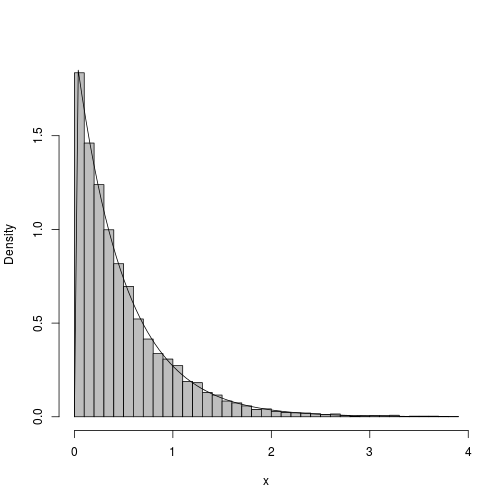(Visited 1,207 times, 1 visits today)

5 thoughts on “Tailoring univariate probability distributions”

1.A.N. Spiess

Nice overview, thanks a lot!
How about a vectorized version of your random sampler, using the vector features of 'runif', 'dexp' and 'ifelse' selection. The only problem is that because the loop is missing, one has to sample more deviates to get the desired N. I noticed that about 92% of samples get rejected, so to sample 100000 random numbers, N = 2E6 will do. And it is about 16 times faster:

my.rexp.reject.vec <- function(N, lambda, max)
{
m <- 12
fxm <- dunif(0, 0, max) * m

# 1. sample from the propsal distribution:
rx <- runif(N, 0, max)
# 2. sample a point along a vertical line
# at the rx point from Uniform(0, f(x)*m):
rv <- runif(N, 0, fxm)
# 3. evaluate the density of p(x) at rx
dx <- dexp(rx, lambda)
# 4. compare and accept if appropriate
samples <- ifelse(rv system.time(x system.time(x length(x)
 166587

>hist(x[1:100000])

Cheers,
Andrej

2.Rick Wicklin

Thanks for this nice summary of the four essential functions for working with statistical distributions. Definitely "must reading" for all statistical programmers.

Because the exponential function is so simple (with analytical CDF and quantile), I wrote a slightly more complicated example that uses the folded normal distribution. Lastly, implementing an efficient acceptance-rejection algorithm in a vector language can be a challenge. I've presented some ideas, and there are more in the comments to my blog. Cheers!

3.Christopher Moore

I just wanted to say thanks for this post! I had been dabbling in and out of doing something similar with a custom pdf and this fit all of the pieces together for me.